# L Systems - creating trees and ferns

By Martin McBride, 2021-06-19
Tags: tree barnsley fern turtle recursion l system
Categories: generativepy generative artIn this post we will look further into L Systems and see how they can be used to tree and fern like structures using generativepy. We will again make use of the simple turtle system provided by generativepy.

## Extending our L System grammar

We will start by creating a simple binary tree like this: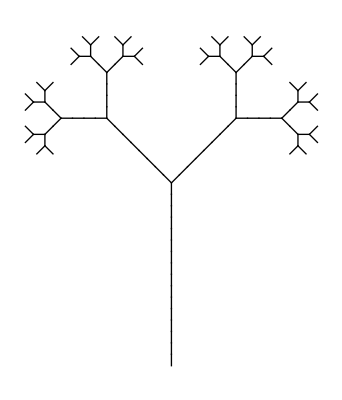This pattern is the 5th generation of a pattern that starts out as a simple Y shape. Unlike simpler L Systems, it exhibits branching. There are 32 "leaves" in the images. Starting from the first generation (a Y shape) which has 2 leaves, the number of leaves doubles on each generation (2 to the power 5 is 32).

To implement branching, we need to add two extra characters to our L System grammar:

• [ doesn't draw anything, but it saves the current turtle position and heading
• ] also doesn't draw anything, but restores the previous turtle position and heading.

So the string [ABC]XYZ saves the initial position then draws ABC (whatever that might be). It then restores the previous position, and draws XYZ starting from the original position and heading. Notice that ABC and XYZ just represent whatever real operations you might want to perform.

The save and restore operations use a stack, so you can save multiple positions, and they will be restored in the reverse order.

## Binary tree parameters

Here are the rules for the binary tree:

F becomes G[+F]-F
G becomes GG
+ becomes +
- becomes -
[ becomes [
] becomes ]


F and G both draw a line length 10. + and - turn left or right by 45 degrees (pi/4).

It is worth looking at the first pass. The initial string F becomes G[+F]-F. Here is what this draws: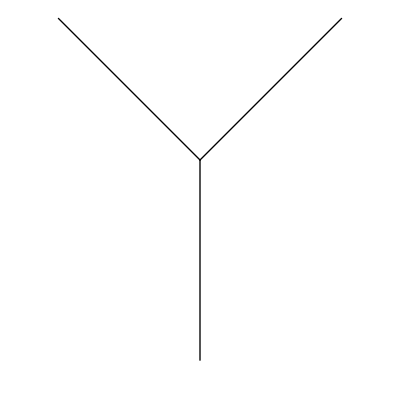Imagine we start of at point A, with the turtle heading upwards.

• G moves 10 units upwards to point B
• [ saves the current position (B) and heading (upwards)
• +F turns the turtle 45 degrees left and draws 10 units to point C
• ] restores the previous position. The turtle is placed back at point B, heading upwards, without drawing anything
• -F turns the turtle 45 degrees right and draws 10 units to point D

Here is the second iteration: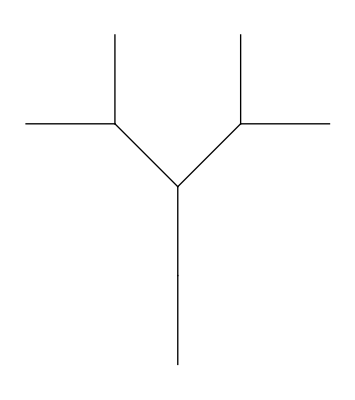This diagram shows the first and second iteration side by side, colour coded to illustrate what is happening: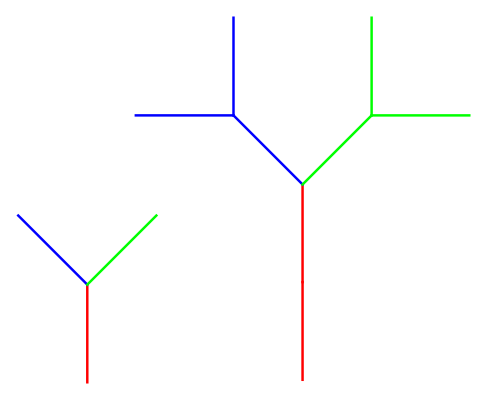• The vertical line (in red) in the first iteration is repalced by two vertcal lines (the rule G becomes GG).
• The left hand diagonal line (in blue) in the first iteration is replaced by a full tree, but rotated by 45 degrees (the rule F becomes G[+F]-F).
• The right hand diagonal line (in red) is also replaced by a full tree in a similar way.

This pattern extends into the 3rd iteration (below) and the 4th iteration (which we saw at the start of the article):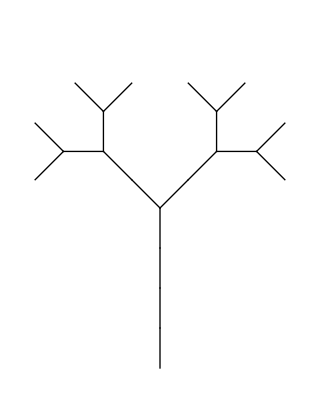We are using different scales to show the iterations, in fact the tree more or less doubles in size on each iteration.

## The code

Here is the binary tree Python code:

from generativepy.drawing import make_image, setup
from generativepy.geometry import Turtle
from generativepy.color import Color
import math

AXIOM = 'F'
RULES = { 'F' : 'G[+F]-F',
'G' : 'GG',
'[' : '[',
']' : ']',
'+' : '+',
'-' : '-' }
ITERATIONS = 6
ANGLE = math.pi/4
LENGTH = 1

ITERATIONS = 1
WIDTH = 2
HEIGHT = 2

def lsystem(start, rules):
out = ''
for c in start:
s = rules[c]
out += s

return out

def draw(ctx, pixel_width, pixel_height, frame_no, frame_count):
setup(ctx, pixel_width, pixel_height, startx=-WIDTH/2, starty=-HEIGHT+WIDTH/10, height=HEIGHT, background=Color(1))

s = AXIOM
for i in range(ITERATIONS):
s = lsystem(s, RULES)

turtle = Turtle(ctx)
turtle.set_style(line_width=HEIGHT/300)
turtle.move_to(0, 0)
turtle.left(math.pi/2)
for c in s:
if c == 'F':
turtle.forward(LENGTH)
if c == 'G':
turtle.forward(LENGTH)
elif c == '[':
turtle.push()
elif c == ']':
turtle.pop()
elif c == '+':
turtle.left(ANGLE)
elif c == '-':
turtle.right(ANGLE)

make_image("lsystem-tree-1.png", draw, int(400*WIDTH/HEIGHT), 400)


The main things to note here are:

• The page is offset by (-WIDTH/2, starty=-HEIGHT+WIDTH/10), so that the initial position of the turtle is near the centre-bottom of the canvas.
• The turtle and turned to point upwards before the shape is drawn..
• We have added the [ and ] cases using the existing Turtle methods push and pop.

## A more realistic plant

We will modify the previous code slightly to create a more realistic looking L System plant.

The first rule is changed to:

F becomes G+[[F]-F]-G[-GF]+F


and the angle is changed to 20 degrees. This gives the following basic shape (ie the shape after 1 iteration):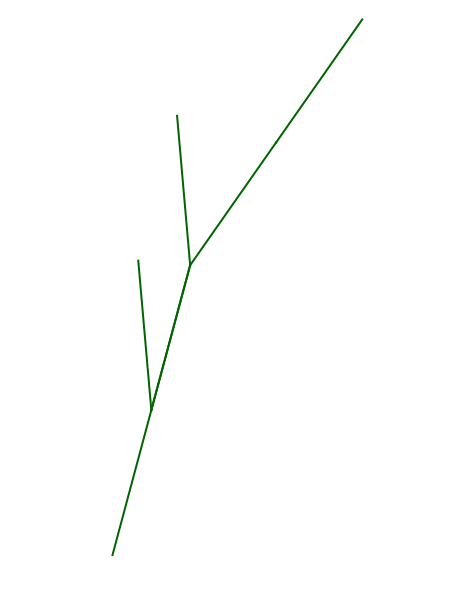You can follow this through, step by step, like we did for the binary tree, if you wish. The shape creates after 6 iterations is: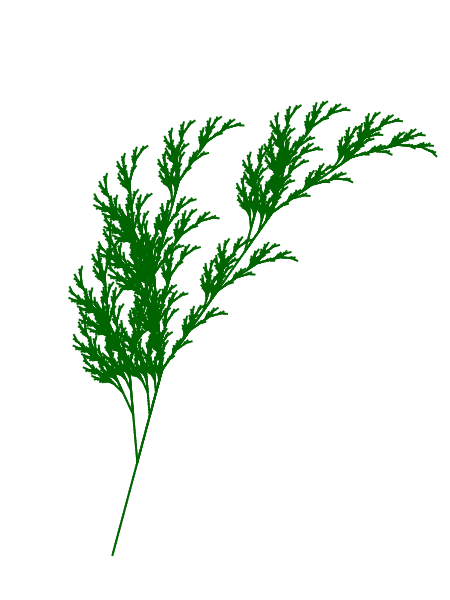The final code is below. All that has really changed are the rules and angle, a bit of adjustment of the size and position, and all importantly making the plant green instead of black.

from generativepy.drawing import make_image, setup, make_svg
from generativepy.geometry import Turtle
from generativepy.color import Color
import math

AXIOM = 'F'
AXIOM = 'F'
RULES = { 'F' : 'G+[[F]-F]-G[-GF]+F',
'G' : 'GG',
'[' : '[',
']' : ']',
'+' : '+',
'-' : '-' }
ITERATIONS = 6
ANGLE = 20*math.pi/180
LENGTH = 1
HEIGHT = 200
WIDTH = 150

def lsystem(start, rules):
out = ''
for c in start:
s = rules[c]
out += s

return out

def draw(ctx, pixel_width, pixel_height, frame_no, frame_count):
setup(ctx, pixel_width, pixel_height, startx=-WIDTH/4, starty=-HEIGHT+WIDTH/10, height=HEIGHT, background=Color(1))

s = AXIOM
for i in range(ITERATIONS):
s = lsystem(s, RULES)

turtle = Turtle(ctx)
turtle.set_style(color=Color('darkgreen'), line_width=HEIGHT/300)
turtle.move_to(0, 0)
turtle.left(75*math.pi/180)
for c in s:
if c == 'F':
turtle.forward(LENGTH)
if c == 'G':
turtle.forward(LENGTH)
elif c == '[':
turtle.push()
elif c == ']':
turtle.pop()
elif c == '+':
turtle.left(ANGLE)
elif c == '-':
turtle.right(ANGLE)

make_image("lsystem-fern.png", draw, 450, 600)